# SAS/ETS Examples

## Bivariate Granger Causality Test

Contents | SAS Program

# Overview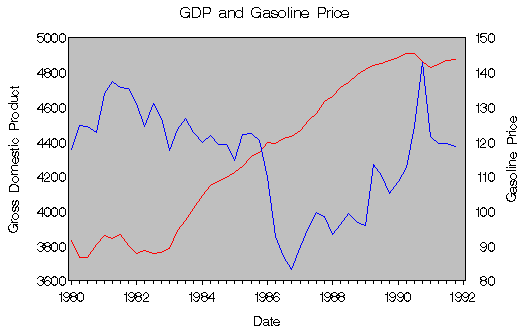Figure 1: GDP and Gasoline Price

A question that frequently arises in time series analysis is whether or not one economic variable can help forecast another economic variable. For instance, it has been well documented that nearly all of the postwar economic recessions have been preceded by large increases in the price of petroleum. Does this imply that oil shocks cause recessions?

One way to address this question was proposed by Granger (1969) and popularized by Sims (1972). Testing causality, in the Granger sense, involves using F-tests to test whether lagged information on a variable Y provides any statistically significant information about a variable X in the presence of lagged X. If not, then "Y does not Granger-cause X."

There are many ways in which to implement a test of Granger causality. On particularly simple approach uses the autoregressive specification of a bivariate vector autoregression. Assume a particular autoregressive lag length p, and estimate the following unrestricted equation by ordinary least squares (OLS):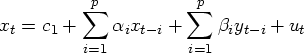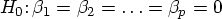Conduct an F-test of the null hypothesis by estimating the following restricted equation also by OLS:
Compare their respective sum of squared residuals.
If the test statistic
is greater than the specified critical value, then reject the null hypothesis that Y does not Granger-cause X.

It is worth noting that with lagged dependent variables, as in Granger-causality regressions, the test is valid only asymptotically. An asymptotically equivalent test is given by
Another caveat is that Granger-causality tests are very sensitive to the choice of lag length and to the methods employed in dealing with any non-stationarity of the time series.

# Analysis

Producing the desired test statistics requires some preliminary data manipulation. Two Citibase data sets are read from the SASHELP library: CITIQTR, from which the variables DATE and GDPQ are kept, and CITIMON, from which DATE and EEGP are kept.

   data gdp;
set sashelp.citiqtr;
keep date gdpq;
run;

data gp;
set sashelp.citimon;
keep date eegp;
run;


A problem arises from the fact that GDPQ is quarterly gross domestic product, measured in billions of 1987 dollars from 1980:1 to 1991:4, while EEGP is an index, with base year 1987, of monthly retail gas prices from January 1980 to December 1991. In order to use these two series in this analysis, it is necessary for the observations to be of the same frequency. The EXPAND procedure can be used to transform the monthly gas prices observations into quarterly observations and merge them with the GDP data to create a combined data set with quarterly observations. The OBSERVED= option enables you to control the observation characteristics of the input time series and of the output series. In this example, the average of three monthly observations are used to create each quarterly observation. Then, the data are lagged two periods using the LAG function.

   proc expand data=gp out=temp from=month to=qtr;
convert eegp / observed=average;
id date;
run;

data combined;
merge gdp temp;
by date;
run;

data causal;
set work.combined;

gdpq_1 = lag(gdpq);
gdpq_2 = lag2(gdpq);

eegp_1 = lag(eegp);
eegp_2 = lag2(eegp);
run;


After the data are processed, the unrestricted and restricted models are estimated using the AUTOREG procedure and output files are created for the residuals for each regression.

   *   unrestricted model;
proc autoreg data=causal;
model gdpq = gdpq_1 gdpq_2 eegp_1 eegp_2;
output out=out1 r=e1;   /* output residuals */
run;

*   restricted model;
proc autoreg data=out1;
model gdpq = gdpq_1 gdpq_2;
output out=out2 r=e0;    /* output residuals */
run;


These residuals can then be read into vectors in PROC IML and used to calculate the test statistics with matrix algebra.

   ods select Iml._LIT1010
Iml.TEST1_P_VAL1
Iml.TEST2_P_VAL2;

ods html body='exgran01.htm';

*   compute test;
proc iml;

start main;

use out1;
close out1;

use out2;
close out2;

p = 2;           /* # of lags         */
T = nrow(e1);    /* # of observations */

sse1 = ssq(e1);
sse0 = ssq(e0);

* F test;
test1 = ((sse0 - sse1)/p)/(sse1/(T - 2*p - 1));
p_val1 = 1 - probf(test1,p,T - 2*p - 1);

* asymtotically equivalent test;
test2 = (T * (sse0 - sse1))/sse1;
p_val2 = 1 - probchi(test2,p);

print "IML Result",, test1 p_val1,,
test2 p_val2;
finish;
run;
quit;

ods html close;


 IML Result

test1 p_val1
3.8623494 0.0286651

test2 p_val2
8.6229196 0.013414

Figure 2: Bivariate Granger Causality Test Results

As shown in Figure 2, with p (the number of lags included in the regressions) set equal to two, both test statistics are significant at the 5% level. Thus, it would seem that past values of petroleum prices help to predict GDP.

# References

Ashley, R. (1988), "On the Relative Worth of Recent Macroeconomic Forecasts," International Journal of Forecasting, 4, 363-376.

Ashley, R., Granger, C.W.J., and Schmalensee, R. (1980), "Advertising and Aggregate Consumption: An Analysis of Causality," Econometrica, 48, 1149-1168.

Berndt, E. (1991), The Practice of Econometrics: Classic and Contemporary, New York: Addison-Wesley.

Geweke, J., Meese, R., and Dent, W. (1983), "Comparing Alternative Tests of Causality in Temporal Systems: Analytic Results and Experimental Evidence," Journal of Econometrics, 21, 161-194.

Granger, C.W.J. (1969), "Investigating Causal Relations by Econometric Methods and Cross-Spectral Methods," Econometrica, 34, 424-438.

Hamilton, J. (1994), Time Series Analysis, Princeton, NJ: Princeton University Press.

Sims, C. (1972), "Money, Income and Causality," American Economic Review, 62, 540-552.

Sims, C. (1980), "Macroeconomics and Reality," Econometrica, 48, 1-48.